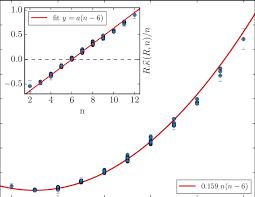## How to Calculate and Solve for Mean Bubble Point in a Fluid | The Calculator EncyclopediaThe image above represents mean bubble point.

To compute for the mean bubble point, one essential parameter is needed and the parameter is Glass gas solubility parameter (x).

The formula for calculating mean bubble point:

Pb* = 10x

Where:

Pb* = Mean Bubble Point
x = Glass Gas Solubility Parameter

Let’s solve an example;
Given that the glass gas solubility parameter is 12. Find the mean bubble point?

This implies that;

x = Glass Gas Solubility Parameter = 12

Pb* = 10x
Pb* = 1012
Pb* = 1000000000000

Therefore, the Mean bubble point is 1000000000000.